# Probability of Fair coin tossed

ecesiu

## Homework Statement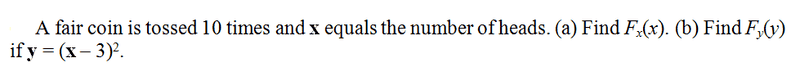## Homework Equations

My solution for part B is different than the manual solution , here is the Manual solution :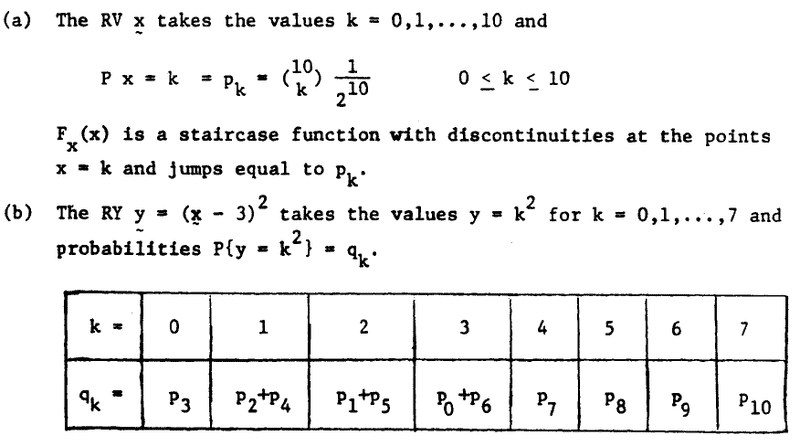## The Attempt at a Solution

and this is my solution :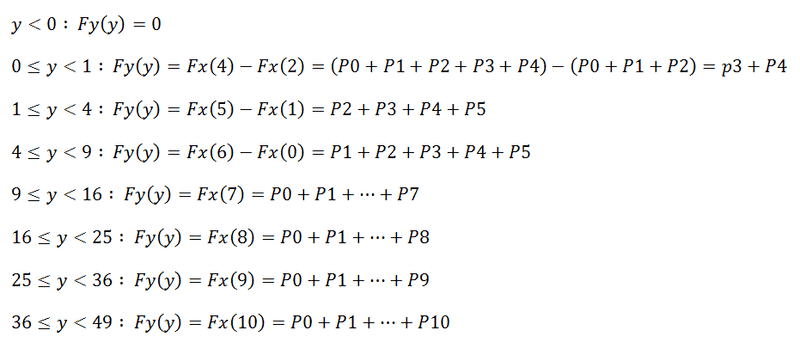Homework Helper
Dearly Missed

## Homework Statement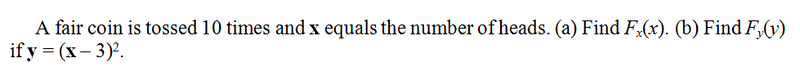## Homework Equations

My solution for part B is different than the manual solution , here is the Manual solution :## The Attempt at a Solution

and this is my solution :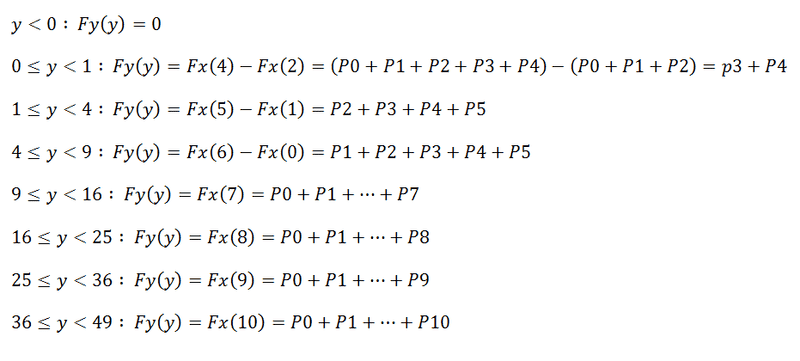For 0 ≤ y < 1, you must exclude the value y = 1, so you just have y = 0; that is, (x-3)^2 = 0, or x = 3. Similarly for the other values.

RGV